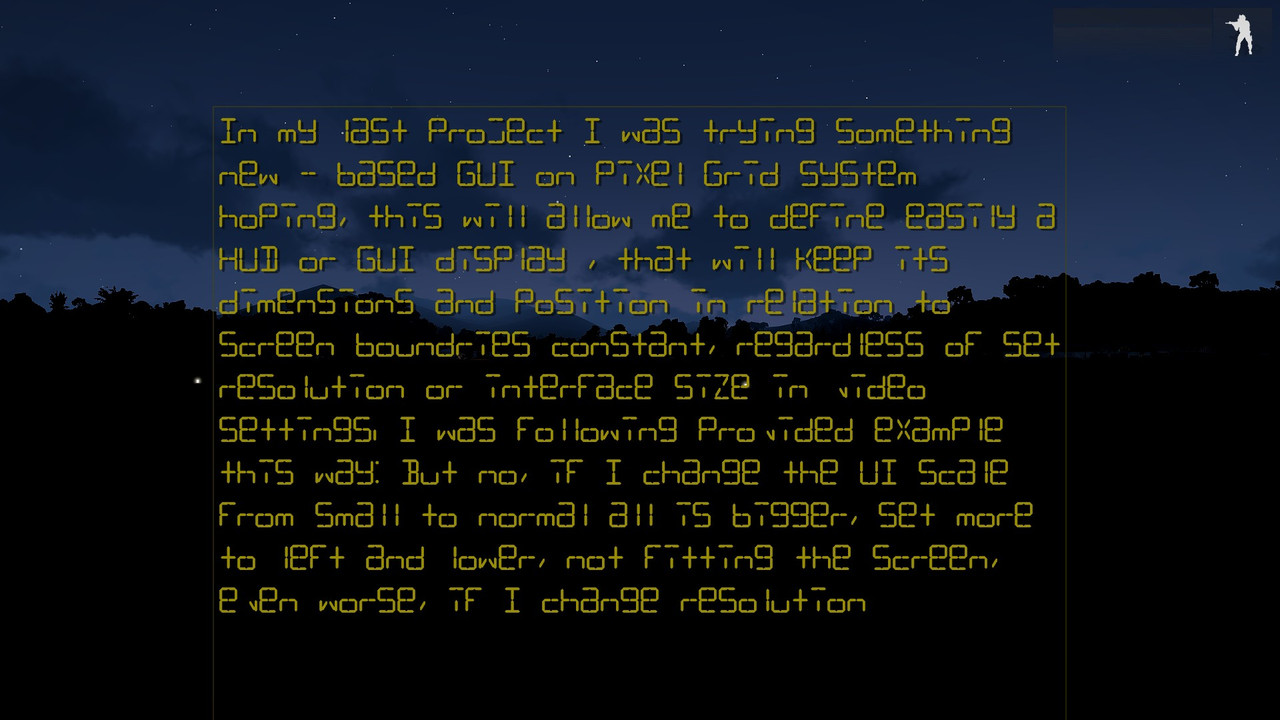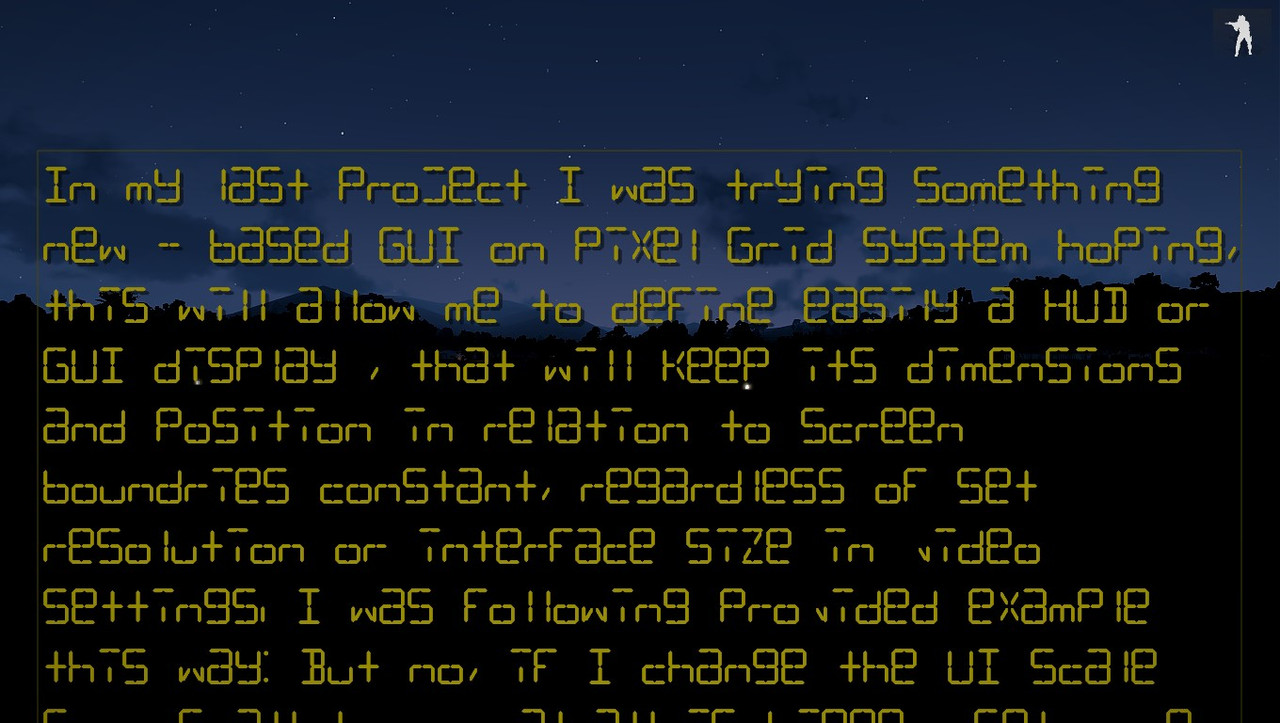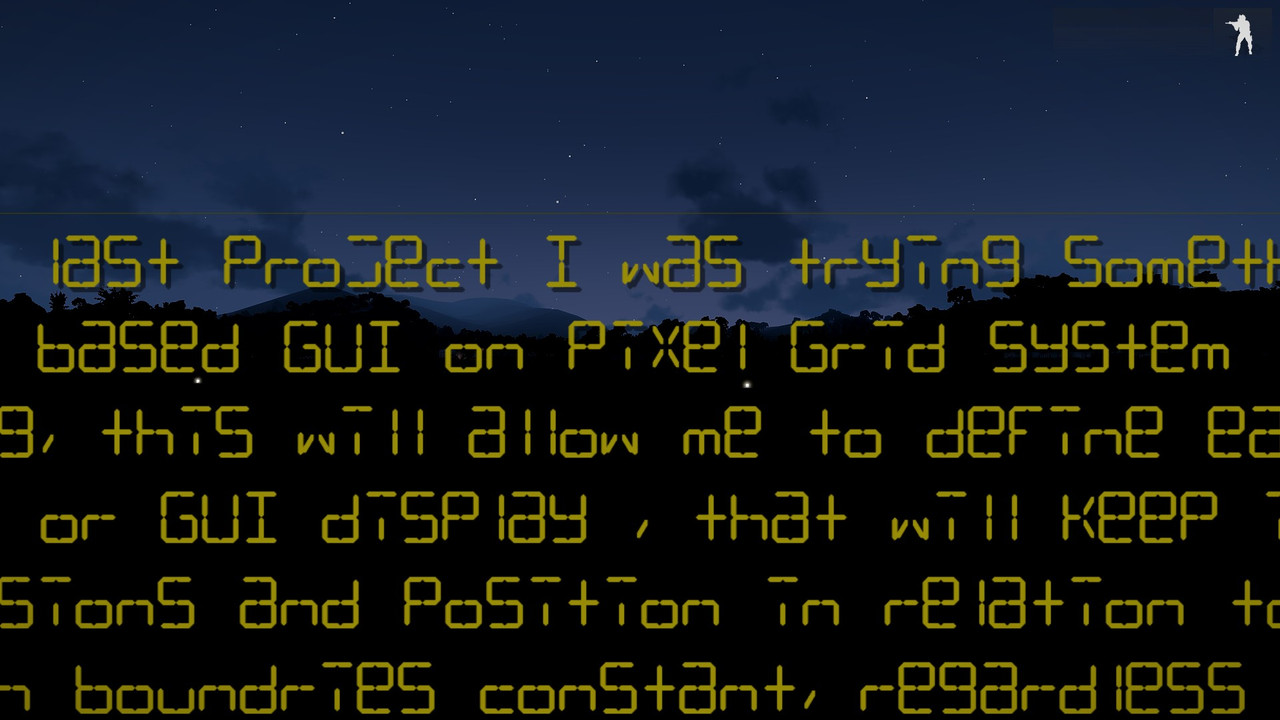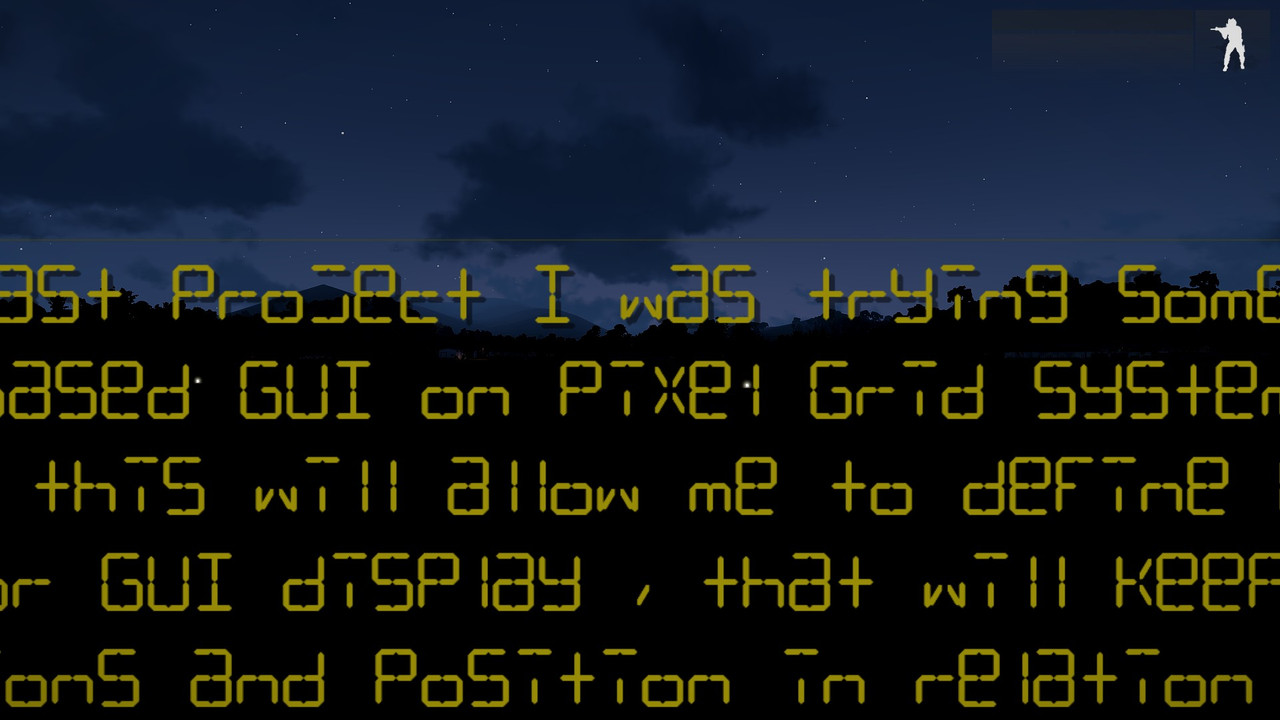# Pixel Grid System, GUI size/position immune to UI scaling and resolution - how?

## Recommended Posts

In my last project I was trying something new - based GUI on Pixel Grid System hoping, this will allow me to define easily a HUD or GUI display , that will keep its dimensions and position in relation to screen boundries constant, regardless of set resolution or interface size in video settings. I was following provided example this way:

Spoiler

```
#define UI_GRID_W (pixelW * pixelGrid) // One grid width
#define UI_GRID_H (pixelH * pixelGrid) // One grid height
#define UI_GUTTER_W (pixelW * 2)       // One “gutter” width
#define UI_GUTTER_H (pixelH * 2)       // One “gutter” height

class DT_RscText
{
x = 0;
y = 0;
h = 0.037;
w = 0.3;
type = 0;
style = 0;
font = "PuristaMedium";
text = "";
lineSpacing = 1;
SizeEx = 0.03;
ColorBackground[] = {0,0,0,0};
ColorText[] = {1,1,1,1};
colorShadow[] = {0, 0, 0, 0.5};
};

//then for example:

class RscIntroText
{
IDD = 260002;
name= "IT";
MovingEnable = 0;
enableSimulation = true;

class controls
{
class RscText_IntroT: DT_RscText
{
idc = 1;
style = 16+512;
font = "LCD14";
x = safeZoneX + (safeZoneW/2) - UI_GRID_W * 80;
y = safeZoneY + UI_GRID_H * 20;
w = UI_GRID_W * 160;
h = UI_GRID_H * 120;
text = "";
sizeEx = 8 * UI_GRID_H;
size = 8 * UI_GRID_H;
colorText[] = {0.6,0.55,0.02,1};
colorBackground[] = {0.0075,0.6,0.075,0};
};
}
};

```

But no, if I change the UI scale from "small" to "normal" all is bigger, set more to left and lower, not fitting the screen, even worse, if I change resolution from 1920x1080 to lower. Using pixelGridNoUIScale or pixelGridBase instead of pixelGrid makes the size difference even bigger. Most likely I misunderstood something. How to achieve, what I try to do? If I define HUD element just below the upper edge of the screen, I do not want it to go out of screen boundary for lower resolution (or farther from the edge for higher resolution) etc.

##### Share on other sites

just to be sure: you did restart the game after changing interface size or screen resolution?

also posting screenshots could help

that said i still use the old school A2/OA approach:

```        x = "(2/100) * SafeZoneW + SafeZoneX";
y = "(76.5/100) * SafeZoneH + SafeZoneY";
w = "(50/100) * SafeZoneW";
h = "(3/100) * SafeZoneH";
size = "0.025 / (getResolution select 5)";
sizeEx = "0.025 / (getResolution select 5)";```

•1

##### Share on other sites

Yes, restarted. I wanted to try new system. They said: By combining the pixelW and pixelH commands with pixelGrid, it is possible to configure displays that will scale correctly with resolutions and interface sizes. Which I understood, the GUI/HUD element will not turn weird as for dimentions and position at change of resolution or interface size. But it does. Screenshots (1:1 fullscreen) using above example with some long text, game restarted between each:

1. pixelGrid:

a) 1920x1080, interface "small" (reference, OK here, as intended, just added the frame around (style = 16;//+512;) for better comparison, yes it is going through lower edge of the screen, that's all right):

Spoilerb) 1920x1080, interface "normal"

Spoilerc) 1360x768, interface "small"

Spoiler2. pixelGridNoUIScale:

a) 1920x1080, "small"

Spoilerb) 1920x1080 "normal" (almost same, as above, moved by few pixels)

Spoilerc) 1360x768, interface "small"

Spoiler3. pixelGridBase:

a) 1920x1080 "small"

Spoilerb) 1920x1080, "normal"

Spoilerc) 1360x768, interface "small"

Spoiler##### Share on other sites

As for the HUD defined in the same manner... Back to the pixelGrid (seems the most right choice after all):

a) 1920x1080, "small" (intended look, centered, at the top)

Spoilerb) 1920x1080, "normal" (I don't know, perhaps it should be that way. I set bigger interface and got HUD bigger, logical, position is OK, but how to make it same size despite larger interface then? Old ways only?):

Spoilerc) 1360x768, "small" (wanted to be same, as a), but resembles b), lines not intended, but that's another matter (controls overlap slightly)):

SpoilerSo, positioning kinda works, dimensions are different though.

•1

##### Share on other sites

Perhaps figured it out just by looking on my own examples. If I'm using pixelGrid, display gets bigger if I change resolution or interface size (b and c larger than a). In the second approach, pixelGridNoUIScale, it gets bigger only after resolution change down (a nad b nearly identical, c larger). pixelGridBase gives same dimensions in all three circumstancies (slight position difference for c). So, I should use the last one. I was misled by the fact, after simple change pixelGrid -> pixelGridBase it turns in all cases much bigger, than it was. So perhaps I just need to adjust the size of the grid by some constant number to scale it down... Now seems clear, how I should understand descriptions of these commands. Need to test that...

##### Share on other sites

personally I like pixel grid more. I used to make UIs in absolute values and then run the config through a converter to output in safezone values but pixel grid macros are way easier to use.

For you aligning the hud with the edge of the screen you should still use safezone values. This example creates the UI from center position so it won't fall out of alignment as you make ur UI size bigger.

Spoiler
```
#define pixelScale 0.5

#define GRID_W (pixelW*pixelGrid*pixelScale)
#define GRID_H (pixelH*pixelGrid*pixelScale)

#define PX_W(n) n*GRID_W
#define PX_H(n) n*GRID_H

#define CENTER_X(n) ((getResolution select 2) * 0.5 * pixelW)-(0.5*(PX_W(n)))
#define CENTER_Y(n) ((getResolution select 3) * 0.5 * pixelH)-(0.5*(PX_H(n)))

class myUI {
idd=1234;

#define DIALOG_W 120
#define DIALOG_H 100
class controls {
class myCtrl {
x=CENTER_X(DIALOG_W);
y=CENTER_Y(DIALOG_H);
w=PX_W(DIALOG_W);
h=PX_H(DIALOG_H);
};
};
};```

•3

##### Share on other sites

That's very nice snippet, thanks a lot. :)

Tried, what I wrote. Yep. Works, tested with two interface sizes and two resolutions.

To close the case properly, for my needs what I must to change in my code, my proper transition values are somewhere very close to 0.44:

#define UI_GRID_W (pixelW * pixelGridBase * 0.44) // One grid width
#define UI_GRID_H (pixelH * pixelGridBase * 0.44) // One grid height

And I have with pixelGridBase what I designed for pixelGrid 1920x1080 small, but this time same no matter, what interface size or resolution, it seems. There are only some one-pixel displacements like these lines on my HUD examples b and c and slightest difference for lower resolution. Both perhaps due to this:

NOTE: It is very important to remember that in order to maintain pixel accuracy, developers must only multiply grid sizes which result in a whole number of pixels. To ensure this, it is best to multiply grids by n / uiScaleFactor. (which I'm not sure, how to apply - what's n and what's uiScaleFactor values - how to put them into my config, meanwhile I'll use workaround, so I'll not need 1 pixel accuracy, no matter of resolution (another, single control as common background to all 6 HUD elements instead to 6 separate backgrounds as tiles)).

•1
•1

##### Share on other sites
On 9/24/2018 at 1:18 PM, Rydygier said:

what's n

The number your multiply pixelGrid# by

On 9/24/2018 at 1:18 PM, Rydygier said:

what's uiScaleFactor

One of the config values that is used to determine the number grids to split your vertical screen space into

`getNumber( configFile >> "uiScaleFactor"  )`
`getNumber( configFile >> "uiScaleMaxGrids"  )`

What the pixelGrid commands represent

```getResolution params[ "", "_resolutionHeight", "", "", "", "_interfaceSize" ];

_uiScaleFactor = getNumber( configFile >> "uiScaleFactor" );
_uiScaleMaxGrids = getNumber( configFile >> "uiScaleMaxGrids" );

//resolution only
_pixelGridBase = _resolutionHeight / _uiScaleMaxGrids;

//resolution and config
_pixelGridNoUIScale = round( _pixelGridBase / _uiScaleFactor ) * _uiScaleFactor;

//resolution, config and interface size
_pixelGrid = round(( _pixelGridNoUIScale * _interfaceSize ) / _uiScaleFactor) * _uiScaleFactor;
```

This is the way im currently designing my UI's

Spoiler
```

#define UISCALEFACTOR getNumber( configFile >> "uiScaleFactor" )

//user defined size of A grid to design your ui around ( integer )
#define GRIDSCALE 8

//Definition for element grid sizes
//Grids are always pixel perfect, you never multiply a grid by anything other than an integer
//If you want smaller grids to design your ui around change the GRIDSCALE
#define GRID_X( NUM ) ( pixelW * pixelGrid * ((( ceil ( NUM )) * ( GRIDSCALE )) / UISCALEFACTOR ))
#define GRID_Y( NUM ) ( pixelH * pixelGrid * ((( ceil ( NUM )) * ( GRIDSCALE )) / UISCALEFACTOR ))

//SafeZone pixel perfect anchor points
#define HORZ_LEFT ( safeZoneX )
#define HORZ_CENTER ( safeZoneX + ( safeZoneW / 2 ))
#define HORZ_RIGHT ( safeZoneX + safeZoneW )
#define VERT_TOP ( safeZoneY )
#define VERT_CENTER ( safeZoneY + ( safeZoneH / 2 ))
#define VERT_BOTTOM ( safeZoneY + safeZoneH )

//Picture element sizes in grids
#define PIC_W 10
#define PIC_H 6

class pixelGridExample {

idd = 10001;

class controls {

//TOP LEFT
class topLeft : ctrlStaticPicture {

x = HORZ_LEFT;
y = VERT_TOP;
w = GRID_X( PIC_W );
h = GRID_Y( PIC_H );

text = "#(rgb,8,8,3)color(1,0,0,1)";
};

//TOP CENTER
class topCenter : topLeft {

x = HORZ_CENTER - GRID_X( PIC_W / 2 );

};

//TOP RIGHT
class topRight : topLeft {

x = HORZ_RIGHT - GRID_X( PIC_W );

};

//CENTER LEFT
class centerLeft : topLeft {

y = VERT_CENTER - GRID_Y( PIC_H / 2 );

};

//CENTER CENTER
class centerCenter : centerLeft {

x = HORZ_CENTER - GRID_X( PIC_W / 2 );

};

//CENTER RIGHT
class centerRight : centerLeft {

x = HORZ_RIGHT - GRID_X( PIC_W );

};

//BOTTOM LEFT
class bottomLeft : topLeft {

y = VERT_BOTTOM - GRID_Y( PIC_H );

};

//BOTTOM CENTER
class bottomCenter : bottomLeft {

x = HORZ_CENTER - GRID_X( PIC_W / 2 );

};

//BOTTOM RIGHT
class bottomRight : bottomLeft {

x = HORZ_RIGHT - GRID_X( PIC_W );

};

};
};
```

This download, although no where near finished so you may get a few script errors from mouse clicks, hopefully can help visualise what is happening. I'm in the process of developing it further to assist in laying out UI's.

Where currently the...

• 'Grid anchor' window provides nine points to safely anchor a UI element to a pixel perfect safeZone value. The Red Square
• ''Grid scale' is your base grid size to design your UI around.
• 'Mouse Position' window shows the full exploded definition for X and Y to the nearest grid position of the mouse cursor.

Again, no where near finished, I'm just providing it as a visualisation tool for this discussion.

•1
•4

##### Share on other sites

How's this going? Still looking forward to it.

•1

##### Share on other sites

Just wanna add another example of a GUI using pixelGrid to this thread. This GUI also uses a controls group. Another thing that is important when working with pixelGrid and pixelW/H is that UI controls can exceed the edge of the monitor screen since we are not defining width and height of the controls as percentage of safeZones.

Spoiler
```
#include "\a3\3DEN\UI\macros.inc"//Eden Editor defines

//Defines for this GUI
#define POS_X_TEXT 1 * GRID_W
#define POS_X_VALUE 25 * GRID_W
#define WIDTH_TEXT 23 * GRID_W
#define WIDTH_VALUE 35 * GRID_W
#define WIDTH_HEADER 59 * GRID_W
#define COLOUR_GREY 0.231,0.254,0.236,1

//Origin X and Y (Makes it easy to adjust the position of the whole GUI)
#define ORIGIN_X  safeZoneX + 6 * GRID_W
#define ORIGIN_Y  safezoneY	+ 18 * GRID_H

//Ignore the sqf code
class Enh_PlacementTools
{
idd = -1;
movingEnable = true;
class ControlsBackground
{
class Background: ctrlStaticBackground
{
x = ORIGIN_X;
y = ORIGIN_Y + 5 * GRID_H;
w = 64 * GRID_W;
h = 90 * GRID_H;
};
{
text = \$STR_ENH_tools_placementTools;
x = ORIGIN_X;
y = ORIGIN_Y;
w = 64 * GRID_W;
h = 5 * GRID_H;
};
class CurrentValue: ctrlEdit
{
idc = 120;
x = ORIGIN_X + 2 * GRID_W;
y = ORIGIN_Y + 88 * GRID_H;
w = 30 * GRID_W;
h = 5 * GRID_H;
canModify = false;
};
class Close: ctrlButtonClose
{
x = ORIGIN_X + 37 * GRID_W;
y = ORIGIN_Y + 88 * GRID_H;
w = WIDTH_TEXT;
h = 5 * GRID_H;
};
};

class Controls
{
class ControlGroup: ctrlControlsGroup
{
x = ORIGIN_X;
y = ORIGIN_Y +  7 * GRID_H;
w = 64 * GRID_W;
h = 80 * GRID_H;
class Controls
{
{
x = POS_X_TEXT;//Inside a controls group absolute values are OK for X and Y, still using pixel precision though
y = 2 * GRID_W;
h = 5 * GRID_H;
colorBackground[] = {COLOUR_GREY};
};
{
x = POS_X_TEXT;
y = 12 * GRID_H;
w = WIDTH_TEXT
h = 5 * GRID_H;
};
{
idc = 10;
x = POS_X_VALUE;
y = 12 * GRID_H;
w = WIDTH_VALUE
h = 5 * GRID_H;
sliderPosition = 20;
sliderRange[] = {0,200};
onSliderPosChanged = "_this call Enh_fnc_placementTools_radius";
};
class InitialAngle: ctrlStatic
{
text = \$STR_ENH_placementTools_initialAngle;
x = POS_X_TEXT;
y = 22 * GRID_H;
w = WIDTH_TEXT
h = 5 * GRID_H;
};
class InitialAngleValue: ctrlXSliderH
{
idc = 20;
x = POS_X_VALUE;
y = 22 * GRID_H;
w = WIDTH_VALUE;
h = 5 * GRID_H;
sliderRange[] = {0,359};
sliderPosition = 0;
onSliderPosChanged = "call Enh_fnc_placementTools_initialAngle";
};
class CentralAngle: ctrlStatic
{
text = \$STR_ENH_placementTools_centralAngle;
x = POS_X_TEXT;
y = 32 * GRID_H;
w = WIDTH_TEXT;
h = 5 * GRID_H;
};
class CentralAngleValue: ctrlXSliderH
{
idc = 30;
x = POS_X_VALUE;
y = 32 * GRID_H;
w = WIDTH_VALUE;
h = 5 * GRID_H;
sliderRange[] = {0,360};
sliderPosition = 360;
onSliderPosChanged = "call Enh_fnc_placementTools_centralAngle";
};
{
x = POS_X_TEXT;
y = 42 * GRID_H;
h = 5 * GRID_H;
colorBackground[] = {COLOUR_GREY};
};
class Spacing: ctrlStatic
{
text = \$STR_ENH_patternTool_spacing;
x = POS_X_TEXT;
y = 52 * GRID_H;
w = WIDTH_TEXT;
h = 5 * GRID_H;
};
class SpacingValue: ctrlXSliderH
{
idc = 40;
x = POS_X_VALUE;
y = 52 * GRID_H;
w = WIDTH_VALUE
h = 5 * GRID_H;
sliderRange[] = {0,50};
sliderPosition = 5;
onSliderPosChanged = "call Enh_fnc_placementTools_spacing";
};
{
x = POS_X_TEXT;
y = 62 * GRID_H;
h = 5 * GRID_H;
colorBackground[] = {COLOUR_GREY};
};
class NumColumns: ctrlStatic
{
text = \$STR_ENH_placementTools_numColumns;
x = POS_X_TEXT;
y = 72 * GRID_H;
w = WIDTH_TEXT;
h = 5 * GRID_H;
};
class NumColumsValue: ctrlXSliderH
{
idc = 50;
x = POS_X_VALUE;
y = 72 * GRID_H;
w = WIDTH_VALUE;
h = 5 * GRID_H;
sliderRange[] = {1,20};
sliderPosition = 2;
onSliderPosChanged = "Enh_PlacementTools_NumColumns = round (_this # 1); call Enh_fnc_placementTools_grid";
};
class SpaceX: ctrlStatic
{
text = \$STR_ENH_placementTools_spacingX;
x = POS_X_TEXT;
y = 82 * GRID_H;
w = WIDTH_TEXT;
h = 5 * GRID_H;
};
class SpaceXValue: ctrlXSliderH
{
idc = 60;
x = POS_X_VALUE;
y = 82 * GRID_H;
w = WIDTH_VALUE
h = 5 * GRID_H;
sliderRange[] = {0,50};
sliderPosition = 5;
onSliderPosChanged = "Enh_PlacementTools_SpaceX = _this # 1; call Enh_fnc_placementTools_grid";
};
class SpaceY: ctrlStatic
{
text = \$STR_ENH_placementTools_spacingY;
x = POS_X_TEXT;
y = 92 * GRID_H;
w = WIDTH_TEXT;
h = 5 * GRID_H;
};
class SpaceYValue: ctrlXSliderH
{
idc = 70;
x = POS_X_VALUE;
y = 92 * GRID_H;
w = WIDTH_VALUE;
h = 5 * GRID_H;
sliderRange[] = {0,50};
sliderPosition = 5;
onSliderPosChanged = "Enh_PlacementTools_SpaceY = _this # 1; call Enh_fnc_placementTools_grid";
};
{
x = POS_X_TEXT;
y = 102 * GRID_H;
h = 5 * GRID_H;
colorBackground[] = {COLOUR_GREY};
};
class A: ctrlStatic
{
text = \$STR_ENH_placementTools_A;
x = POS_X_TEXT;
y = 112 * GRID_H;
w = WIDTH_TEXT
h = 5 * GRID_H;
};
class AValue: ctrlXSliderH
{
idc = 80;
x = POS_X_VALUE;
y = 112 * GRID_H;
w = WIDTH_VALUE;
h = 5 * GRID_H;
sliderRange[] = {1,500};
sliderPosition = 50;
onSliderPosChanged = "Enh_PlacementTools_A = _this # 1; call Enh_fnc_placementTools_fillArea";
};
class B: ctrlStatic
{
text = \$STR_ENH_placementTools_B;
x = POS_X_TEXT;
y = 122 * GRID_H;
w = WIDTH_TEXT
h = 5 * GRID_H;
};
class BValue: ctrlXSliderH
{
idc = 90;
x = POS_X_VALUE;
y = 122 * GRID_H;
w = WIDTH_VALUE;
h = 5 * GRID_H;
sliderRange[] = {1,500};
sliderPosition = 50;
onSliderPosChanged = "Enh_PlacementTools_B = _this # 1; call Enh_fnc_placementTools_fillArea";
};
{
x = POS_X_TEXT;
y = 132 * GRID_H;
h = 5 * GRID_H;
colorBackground[] = {COLOUR_GREY};
};
class AreaSize: ctrlStatic
{
text = \$STR_ENH_placementTools_areaSize;
x = POS_X_TEXT;
y = 142 * GRID_H;
w = WIDTH_TEXT;
h = 5 * GRID_H;
};
class AreaSizeValue: ctrlXSliderH
{
idc = 100;
x = POS_X_VALUE;
y = 142 * GRID_H;
w = WIDTH_VALUE;
h = 5 * GRID_H;
sliderRange[] = {0,500};
sliderPosition = 50;
onSliderPosChanged = "Enh_PlacementTools_AreaDia = _this # 1; call Enh_fnc_placementTools_garrison";
};
class Coverage: ctrlStatic
{
text = \$STR_ENH_placementTools_coverage;
x = POS_X_TEXT;
y = 152 * GRID_H;
w = WIDTH_TEXT;
h = 5 * GRID_H;
};
class CoverageValue: ctrlToolbox
{
x = POS_X_VALUE;
y = 152 * GRID_H;
w = WIDTH_VALUE;
h = 5 * GRID_H;
rows = 1;
columns = 4;
strings[] =
{
"10 %",
"25 %",
"50 %",
"100 %"
};
values[] = {10,4,2,1};
onToolBoxSelChanged  = "params ['_ctrl','_index']; Enh_PlacementTools_Coverage = (_ctrl lbValue _index); call Enh_fnc_placementTools_garrison";
};
{
text = "Rectangle Header";
x = POS_X_TEXT;
y = 162 * GRID_H;
h = 5 * GRID_H;
colorBackground[] = {COLOUR_GREY};
};
class RectangleA: ctrlStatic
{
text = "L1";
x = POS_X_TEXT;
y = 172 * GRID_H;
w = WIDTH_TEXT;
h = 5 * GRID_H;
};
class RectangleAValue: ctrlXSliderH
{
idc = 130;
x = POS_X_VALUE;
y = 172 * GRID_H;
w = WIDTH_VALUE;
h = 5 * GRID_H;
sliderRange[] = {1,200};
sliderPosition = 50;
onSliderPosChanged = "Enh_PlacementTools_RectangleA = round (_this # 1); call Enh_fnc_placementTools_rectangle";
};
class RectangleB: ctrlStatic
{
text = "L2";
x = POS_X_TEXT;
y = 182 * GRID_H;
w = WIDTH_TEXT;
h = 5 * GRID_H;
};
class RectangleBValue: ctrlXSliderH
{
idc = 140;
x = POS_X_VALUE;
y = 182 * GRID_H;
w = WIDTH_VALUE;
h = 5 * GRID_H;
sliderRange[] = {1,200};
sliderPosition = 50;
onSliderPosChanged = "Enh_PlacementTools_RectangleB = round (_this # 1); call Enh_fnc_placementTools_rectangle";
};
class RectangleSpacing: ctrlStatic
{
text = \$STR_ENH_patternTool_spacing;
x = POS_X_TEXT;
y = 192 * GRID_H;
w = WIDTH_TEXT;
h = 5 * GRID_H;
};
class RectangleSpacingValue: ctrlXSliderH
{
idc = 150;
x = POS_X_VALUE;
y = 192 * GRID_H;
w = WIDTH_VALUE;
h = 5 * GRID_H;
sliderRange[] = {1,20};
sliderPosition = 5;
onSliderPosChanged = "Enh_PlacementTools_RectangleSpacing = round (_this # 1); call Enh_fnc_placementTools_rectangle";

};
};
};
};
};```